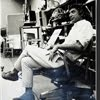## Eugene Ray SDSU 1970'sEugene Ray 1970's. photo credit: Tom Davis

## Tuesday, January 7, 2014

### EVERYTHING IS ENERGY / some visible / some invisible

+VIBRATING ENERGY+
>is at the heart of the issue<
^^^^^^^^^^^^^^^^^
+FORCE FIELDS AFFECT OUR+
+PHYSICAL WELL BEING+
^^^^^^^^^^^^^^^^^^^^
+OPEN MINDS+
>international UFO conference is a fine<
>source of current insight on several<
>fronts next month in arizona<
^^^^^^^^^^^^^^^^^^
+TIMOTHY GOOD+
>brilliant english UFO researcher is on<
>the schedule and not to be missed<
+"ALIEN BASE"+
>is just one of his sixteen highly<
>respected UFO study books<
^^^^^^^^^^^^^^^^^^^
>as is advanced biotronic energy design<
>(the major interests i bring to research)<
^^^^^^^^^^^^^^^^^^^^^^^^^^
>cones & pyramids provide radiant energy<
>phenomina so ancient it is in the future<
+BIOTRONICS+
>(spheres of course also very vital)<
^^^^^^^^^^^^^^^^^^^^^^
>photo of bronze pyramidal grid energy plate<
+INFRARED PHOTO+
>reflects the important work of the seer<
+PATRICK FLANIGAN+
>our friend of 1970s energy research<
^^^^^^^^^^^^^^^^^^^^^^^^^

+ROUND STRUCTURES+
>such as the new orleans residence below<
+SUMMER 1966+
>became my focus in the nineteen sixties<
^^^^^^^^^^^^^^^^^^^^^^^^^^^
>ferro cement roof residence / nola louisiana<
>ten years before my california biomorph<
+FERRO CEMENT+
^^^^^^^^^^^^^^^^^^^^^^^^^^
>radiant concepts / (designing chairs to cities)<
>archaeological dig cover / S.D. presideo<
+DETAIL PHOTO+
>of one of my annual exhibition panels<
^^^^^^^^^^^^^^^^^^^^^^^^^^

+THE SILVER SHIP+
>my studio in lajolla california was the<
>setting of much of the biotronic<
>research and development<
^^^^^^^^^^^^^^^^^
>sun control awnings raise and lower with<
>cables up to the second via third floor<
+SUN AWNINGS+
>using a system of metal pulleys<
^^^^^^^^^^^^^^^^^^^^^
>forty foot long by ten foot high wood truss<
>carries prefab truss joists that bear the<
+STUDIO SPACE+
>second and third floor providing<
>clear, no column or wall space<
^^^^^^^^^^^^^^^^^^^^
>the round parking deck became the symbol<
>of much of my prototype design seen in<
>the ends of the silver ship structure a<
>form of stretched energy tower<
^^^^^^^^^^^^^^^^^^^^^^

+SOLAR TOWERS+
>are designed below in rammed earth and<
>concrete block sandwich walls with<
+PASSIVE HEAT+
>openable dome summer sleeping<
^^^^^^^^^^^^^^^^^^^^^^^
>double passive solar tower prototype<
>to be exhibited at desert incidents<
>"desert incidents" environmental design<
>research development and sales center<
^^^^^^^^^^^^^^^^^^^^^^^^^^

>sdsu art dept mfa poster of nineteen eighties
>our sdsu synergetic media photo lower left<
>sdsu mfa poster of the nineteen eighties<
+1969-1996+
>were my 25 years at SDSU env. design<
^^^^^^^^^^^^^^^^^^^^^^^^^^

+NEW ORLEANS VISIONARY+
>our brother in the vieux carre<
>our older brother, randolph j. ray jr., who<
>was my inspiration for advanced vision<
^^^^^^^^^^^^^^^^^^^^^^^^^^
>DIED AT AGE 39 AND+
+IS MUCH MISSED+
^^^^^^^^^^^^^
eugene ray, mfa, architect
professor emeritus, sdsu